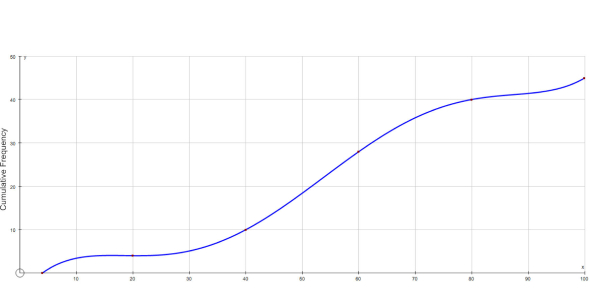# Cumulative Rationals: Operations With Fractions

7 Questions | Total Attempts: 32SettingsCumulative quiz covers material: adding , subtracting, multiplying, and dividing fractions. Quiz also recalls knowledge of receiprocals,improper fractions and mixed numbers, ascentding and descending orders,equivalent fractions,and reduced forms. .

• 1.
Write each  fraction as an equivalent fraction with the given denominator.(1/3)=( _ /15)
• A.

1/15

• B.

5/15

• C.

4/15

• D.

7/15

• E.

3/15

• 2.
Write 17/5 as a mixed number.
• A.

2 and 3/4

• B.

1 and 3/5

• C.

4 and 1/5

• D.

3 and 2/5

• E.

2 and 7/5

• 3.
Which fraction equals 4 and 2/7?
• A.

13/7

• B.

7/13

• C.

7/30

• D.

30/7

• E.

15/7

• 4.
Solve the problem(2/5) + (1/5)=
• A.

5/5

• B.

1/5

• C.

2/10

• D.

3/10

• E.

3/5

• 5.
Solve the problem(2/3)+(4/3)=
• A.

6/9

• B.

8/9

• C.

6/6 or1

• D.

2

• E.

6/9

• 6.
Solve the problem(2/3)+(1/6)=
• A.

5/6

• B.

3/9

• C.

2/18

• D.

5/6

• E.

3/6

• 7.
Solve the problem(7/8)- (3/4)=
• A.

4/4 or 1

• B.

4/8

• C.

1/8

• D.

10/12

• E.

21/32

Related TopicsBack to top